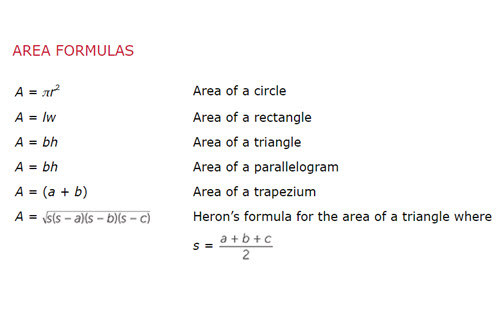Go to website

# Formulae

This module from the AMSI 'The Improving Mathematics Education in Schools (TIMES) project' is for teachers who wish to consolidate their content knowledge on the topic of formulae: what they are, substitution and changing the subject. There are sections that provide history and context for the topic, as well as exercises and examples of how to present and explain the concepts to students.

Year level(s) Year 8, Year 10, Year 9
Purpose Content knowledge
Keywords formula, substitution, changing the subject, explicit teaching

## Curriculum alignment

Strand and focus Algebra
Topics Algebraic expressions
AC: Mathematics (V9.0) content descriptions
AC9M8A02
Graph linear relations on the Cartesian plane using digital tools where appropriate; solve linear equations and one-variable inequalities using graphical and algebraic techniques; verify solutions by substitution

AC9M9A04
Identify and graph quadratic functions, solve quadratic equations graphically and numerically, and solve monic quadratic equations with integer roots algebraically, using graphing software and digital tools as appropriate

AC9M10A01

Expand, factorise and simplify expressions and solve equations algebraically, applying exponent laws involving products, quotients and powers of variables, and the distributive property

Numeracy progression Number patterns and algebraic thinking (P7, P9)